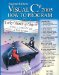# B.4. Converting from Binary, Octal or Hexadecimal to Decimal

We are accustomed to working in decimal, and therefore it is often convenient to convert a binary, octal, or hexadecimal number to decimal to get a sense of what the number is "really" worth. Our diagrams in Section B.1 express the positional values in decimal. To convert a number to decimal from another base, multiply the decimal equivalent of each digit by its positional value and sum these products. For example, the binary number 110101 is converted to decimal 53, as shown in Fig. B.8.

Figure B.8. Converting a binary number to decimal.

Converting a binary number to decimal

Postional values:

32

16

8

4

2

1

Symbol values:

1

1

0

1

0

1

Products:

1*32=32

1*16=16

0*8=0

1*4=4

0*2=0

1*1=1

Sum:

= 32 + 16 + 0 + 4 + 0s + 1 = 53

To convert octal 7614 to decimal 3980, we use the same technique, this time using appropriate octal positional values, as shown in Fig. B.9.

Figure B.9. Converting an octal number to decimal.

Converting an octal number to decimal

Positional values:

512

64

8

1

Symbol values:

7

6

1

4

Products

7*512=3584

6*64=384

1*8=8

4*1=4

Sum:

= 3584 + 384 + 8 + 4 = 3980

To convert hexadecimal AD3B to decimal 44347, we use the same technique, this time using appropriate hexadecimal positional values, as shown in Fig. B.10.

Figure B.10. Converting a hexadecimal number to decimal.

Converting a hexadecimal number to decimal

Postional values:

4096

256

16

1

Symbol values:

A

D

3

B

Products

A*4096=40960

D*256=3328

3*16=48

B*1=11

Sum:

= 40960 + 3328 + 48 + 11 = 44347Visual C# 2005 How to Program (2nd Edition)
ISBN: 0131525239
EAN: 2147483647
Year: 2004
Pages: 600